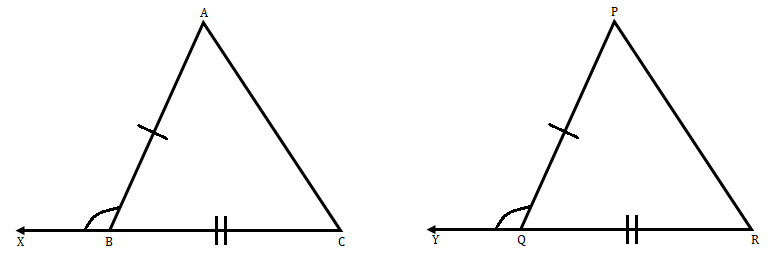"
">

# In ‚ąÜABC and ‚ąÜPQR, AB = PQ, BC = QR and CB and RQ are extended to X and Y respectively and ‚ą†ABX = ‚ą†PQY. Prove that ‚ąÜABC ‚ČÖ ‚ąÜPQR."

Given:

AB = PQ, BC = QR

CB and RQ are extended to X and Y respectively

∠ABX = ∠PQY

To prove: Here we have to prove that ∆ABC ≅ ∆PQR.

Solution:∠ABC $+$ ∠ABX = 180   (Straight angle)   ....(i)

∠PQR $+$ ∠PQY = 180   (Straight angle)   ....(ii)

From (i) and (ii):

∠ABC $+$ ∠ABX = ∠PQR $+$ ∠PQY

Given that, ∠ABX = ∠PQY

∠ABC = ∠PQR       ....(iii)

Now, in ∆ABC and ∆PQR:

AB = PQ                  (Given)

∠ABC = ∠PQR        (from eq. iii)

BC = QR                  (Given)

So, ∆ABC ≅ ∆PQR by SAS criteria.

Updated on: 10-Oct-2022

27 Views